# NCERT Solutions for Class 10 Science Chapter 10: Light Reflection and Refraction

## Ncert Solutions For Class 10 Science Chapter 10 PDF Free Download

The syllabus of NCERT class 10 Science textbook is structured in a way to present a comprehensible coverage of scientific topics related to our daily life. The basic science has been laid out to students with no sharp divisions into disciplines such as Physics, Chemistry and Biology. NCERT is accepted and recommended throughout the schools in the nation. Following are a few reasons to rely on NCERT solutions:

• The tricky questions inserted between chapters force students to think out of the box and apply the concepts learned in the chapter.
• NCERT solutions provide you with detailed solutions to the chapter questions that help you fetch marks in exams.

NCERT solutions are popular among students, especially for science and mathematics. NCERT solutions for class 10 science chapter 10 – Light Reflection and Refraction provided here consist of well-explained solutions to all the questions asked in the textbook. Get your hands on the comprehensive NCERT solutions for chapter 10 Light Reflection and Refraction here, for free!

### Access Chapter wise NCERT Solutions for Class 10 Science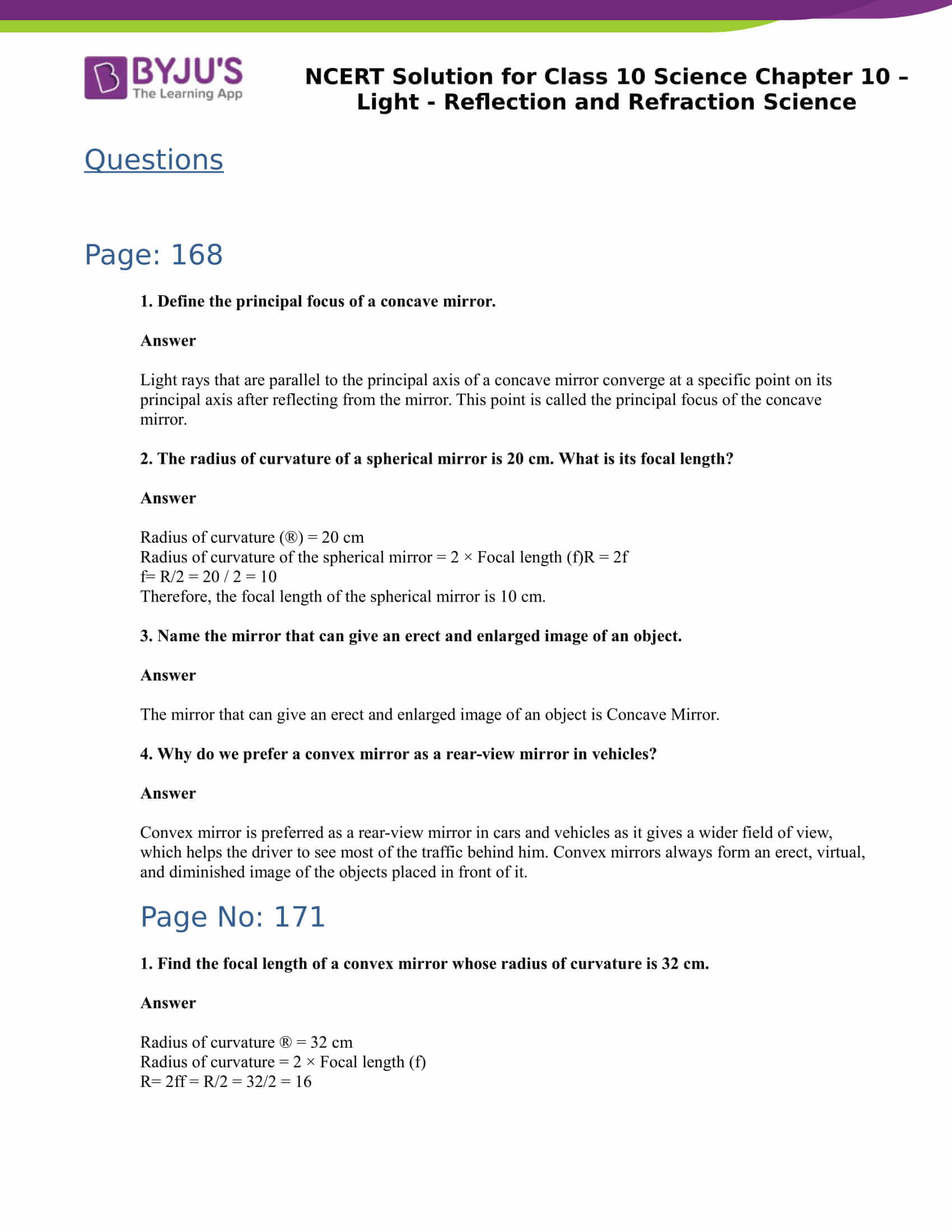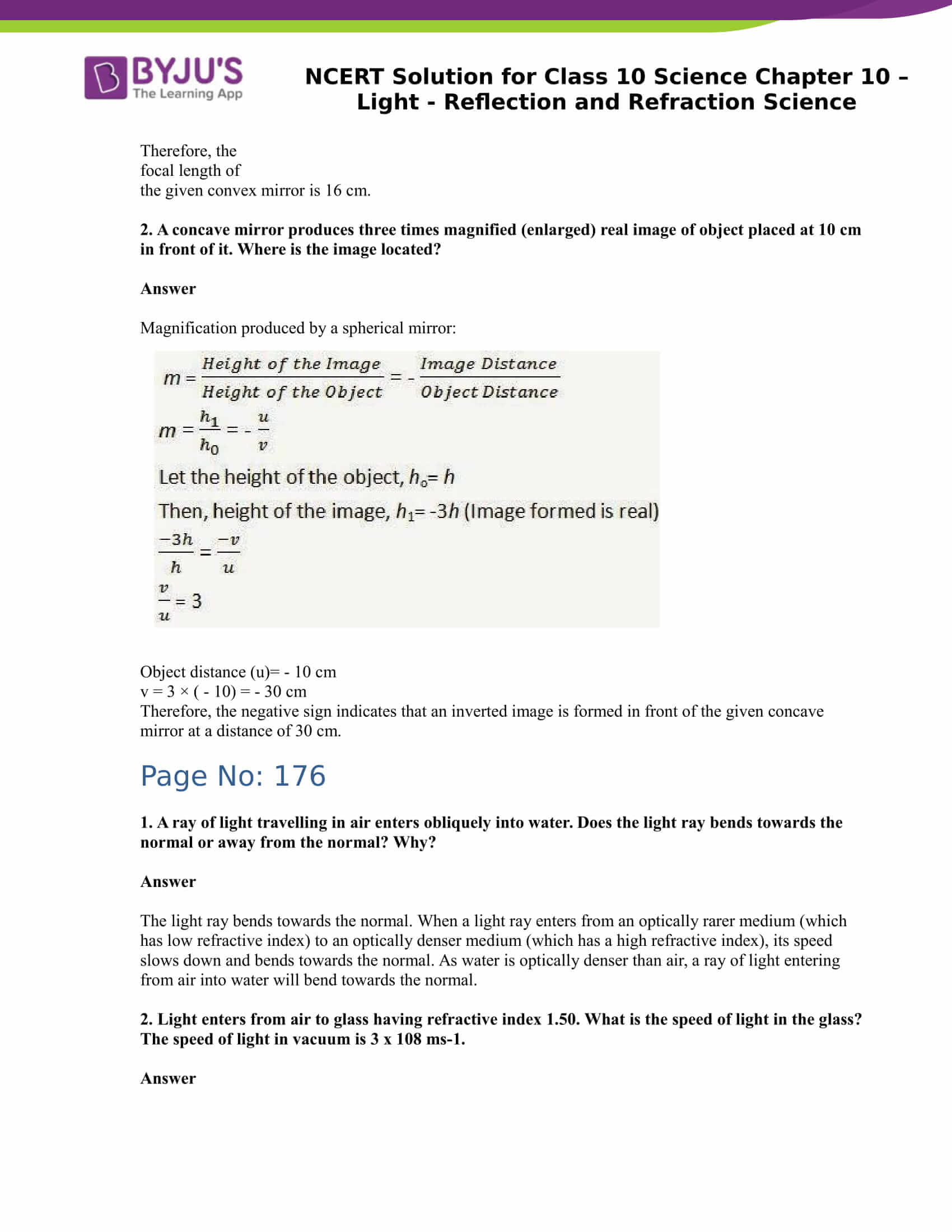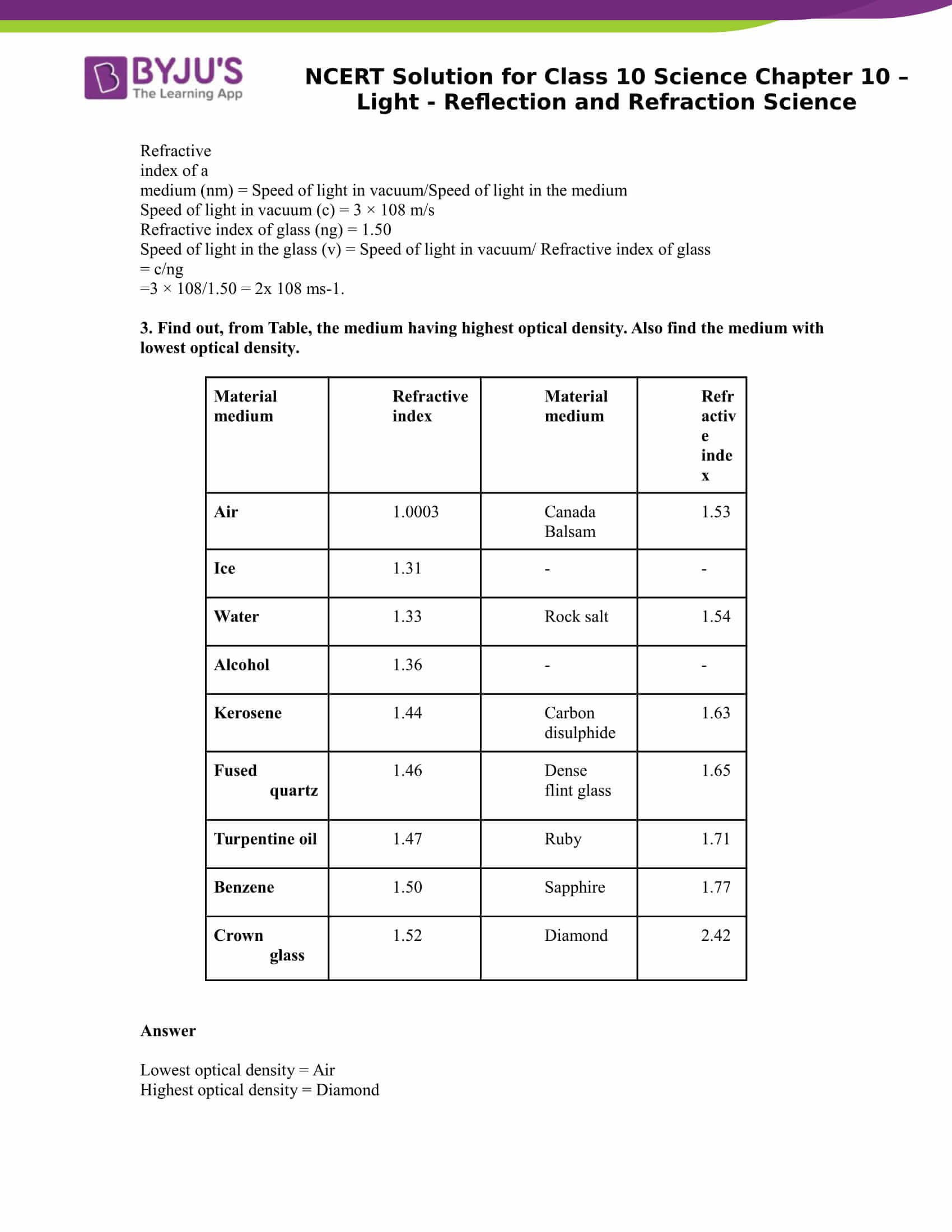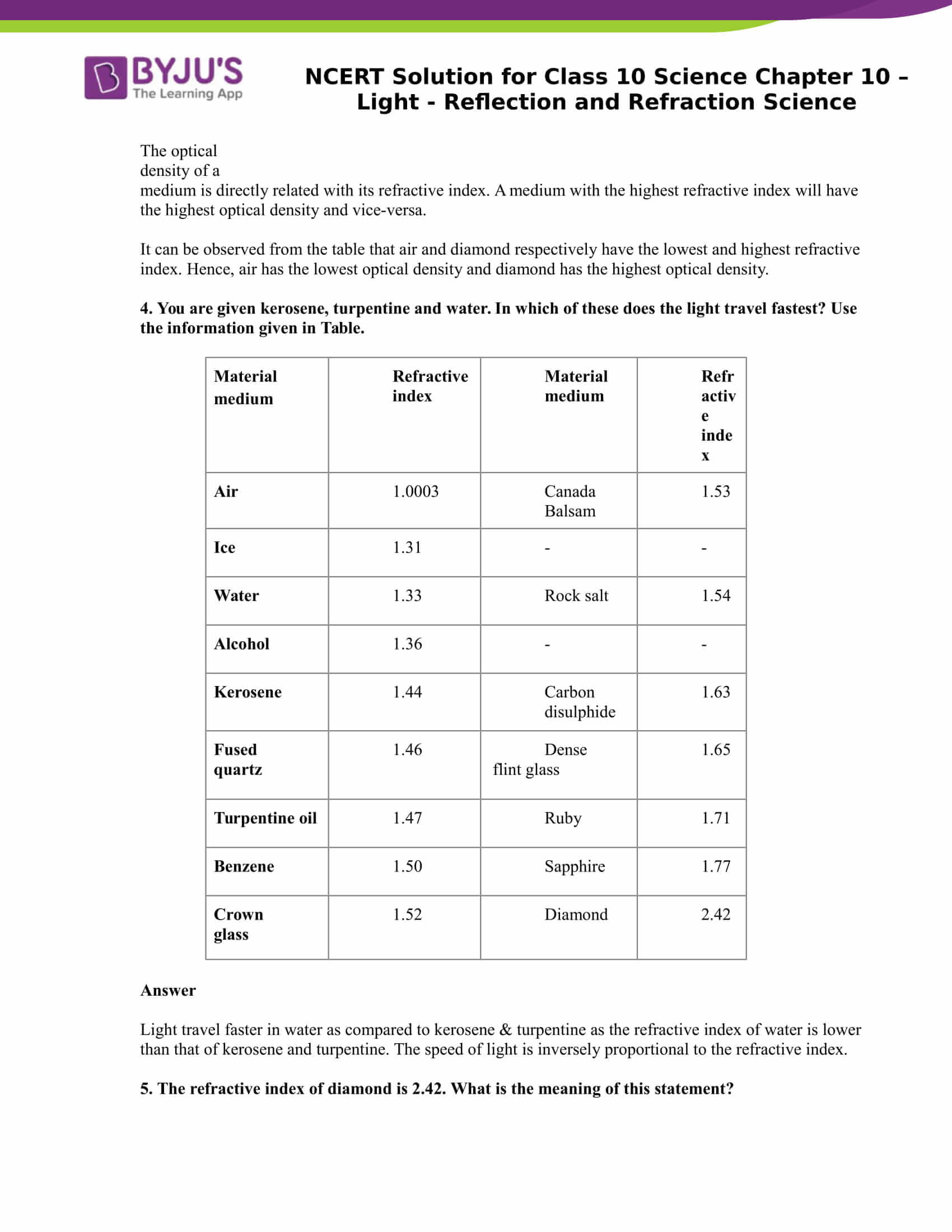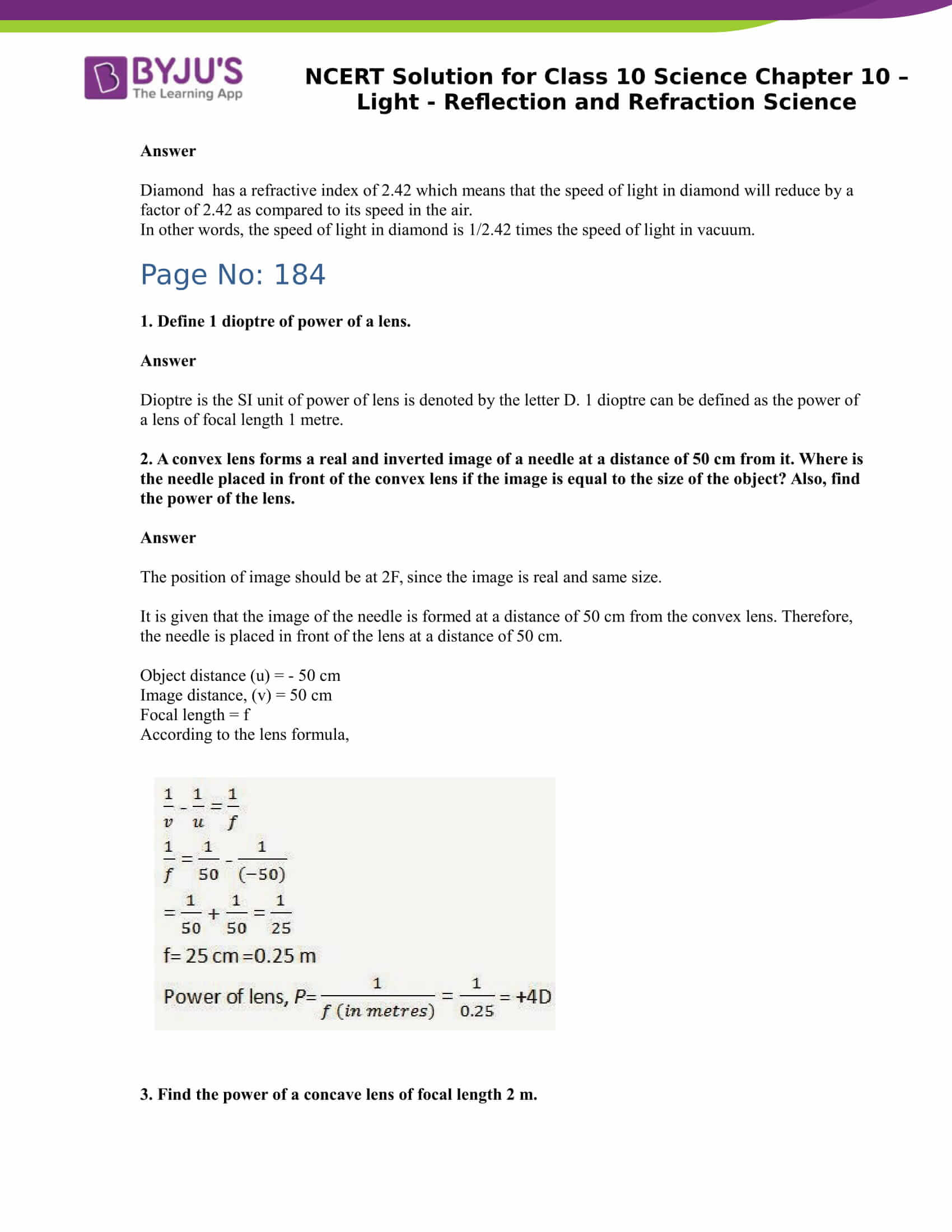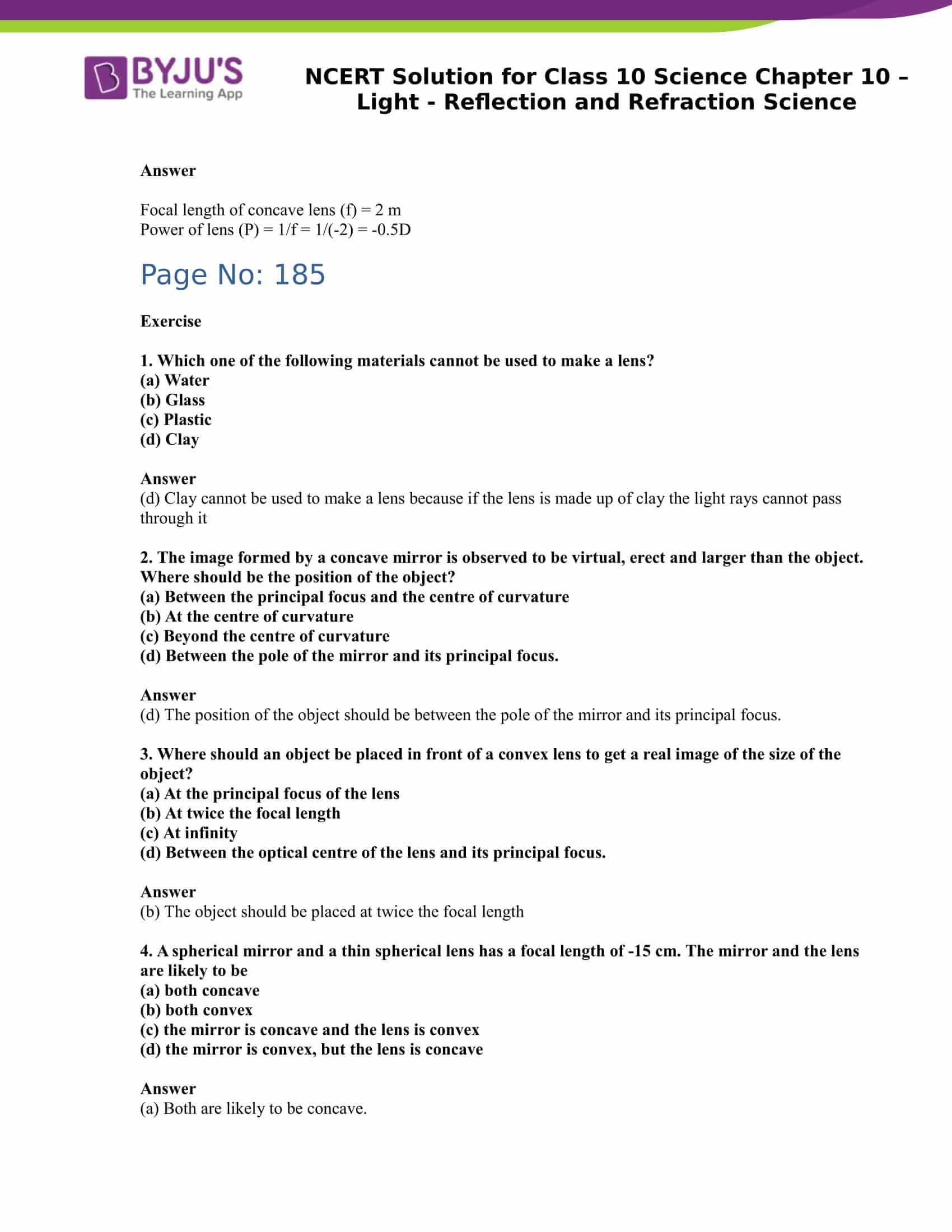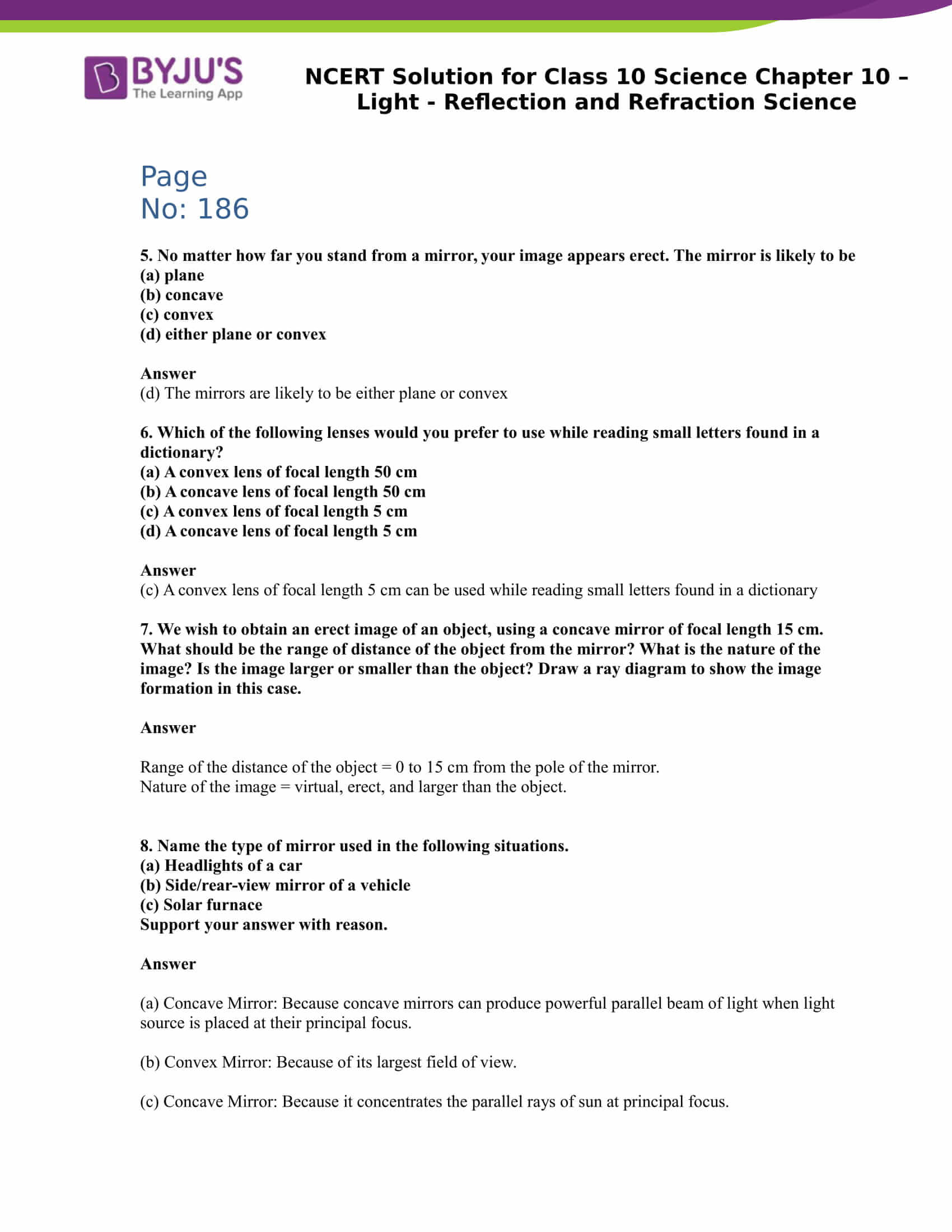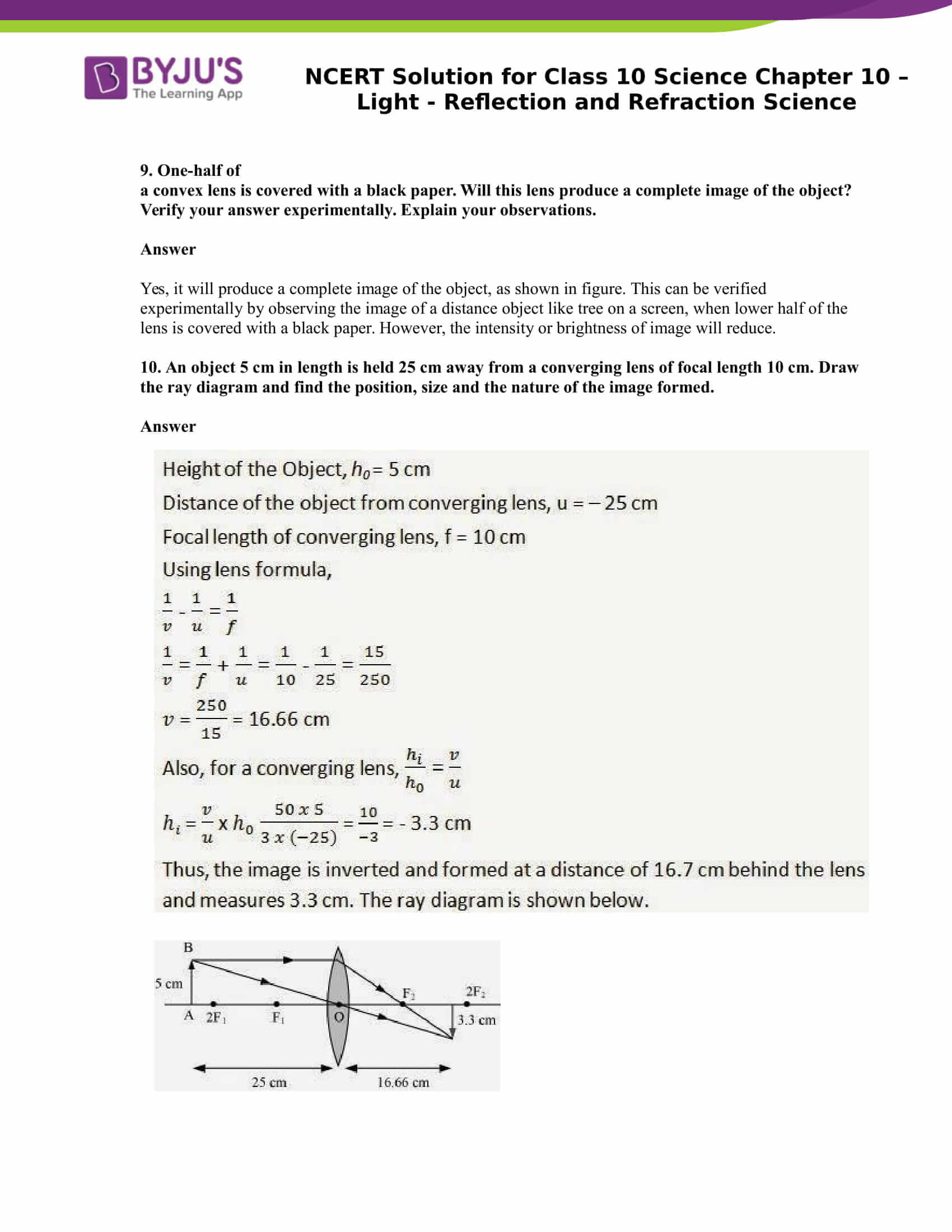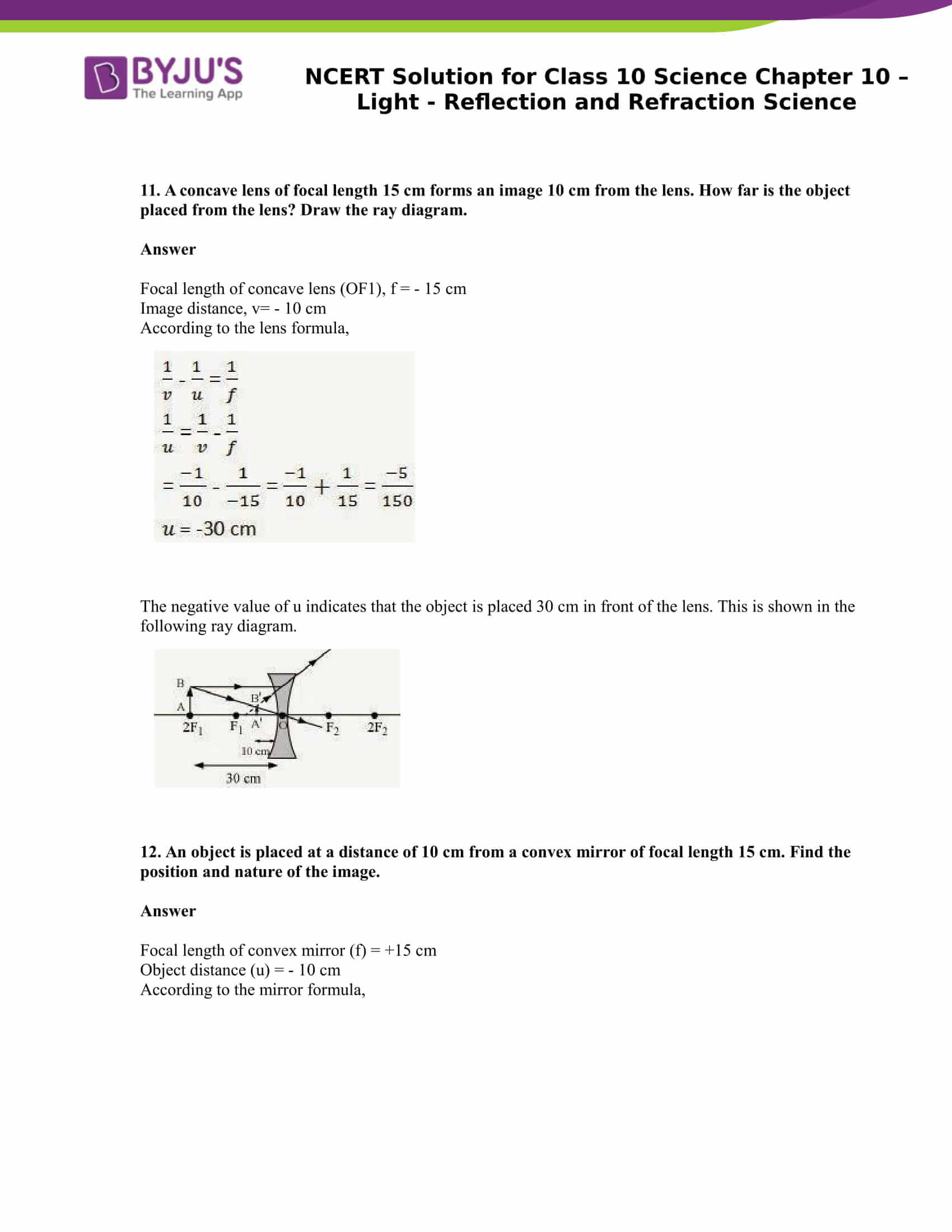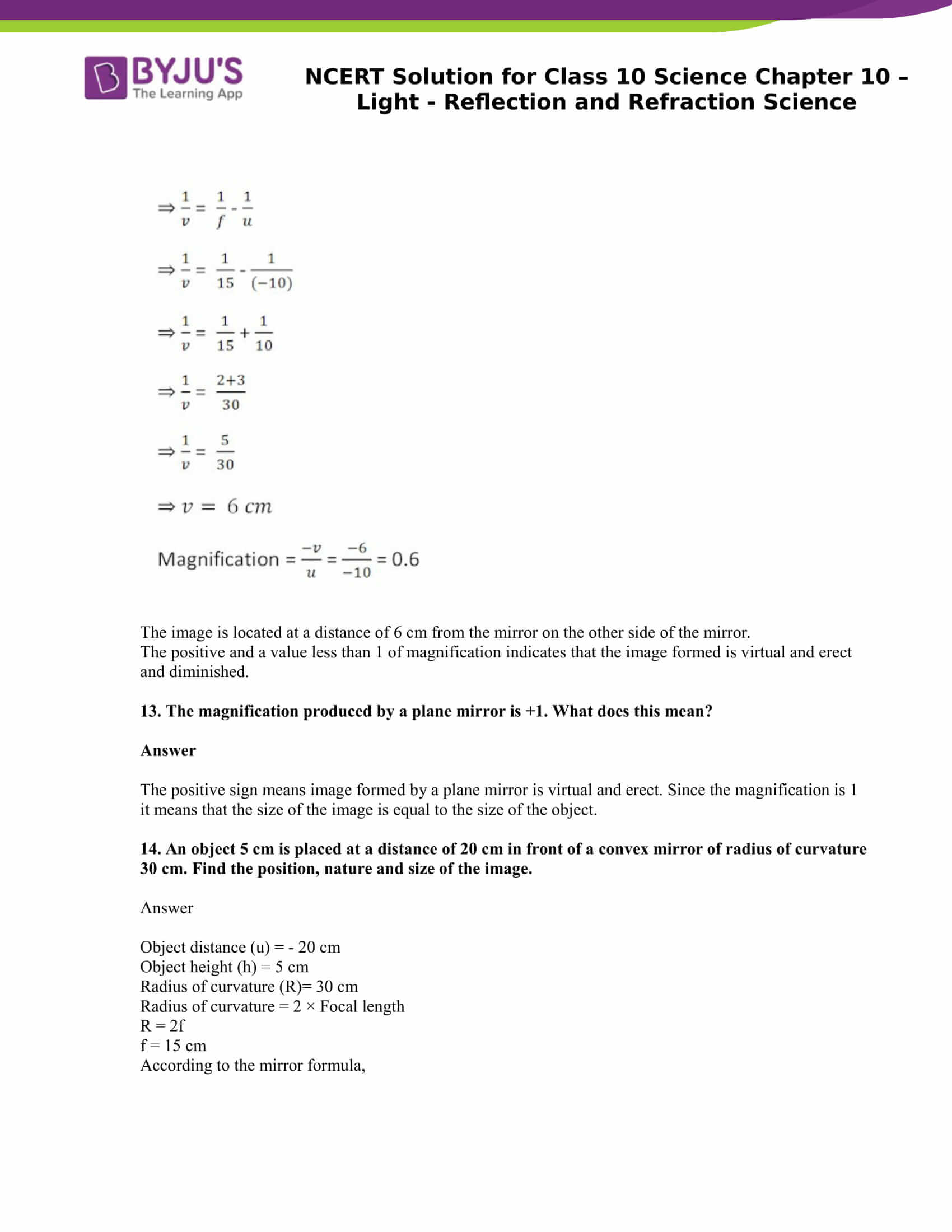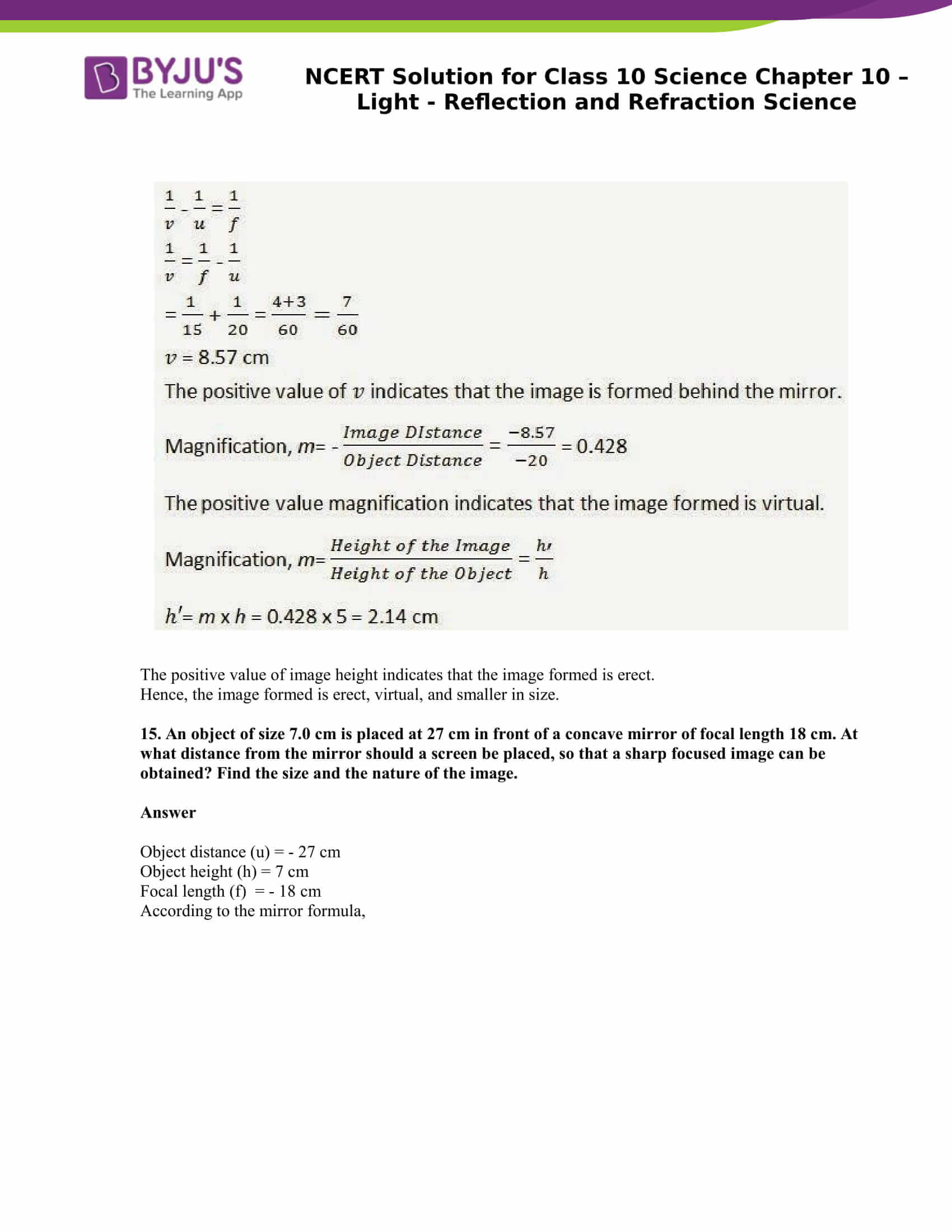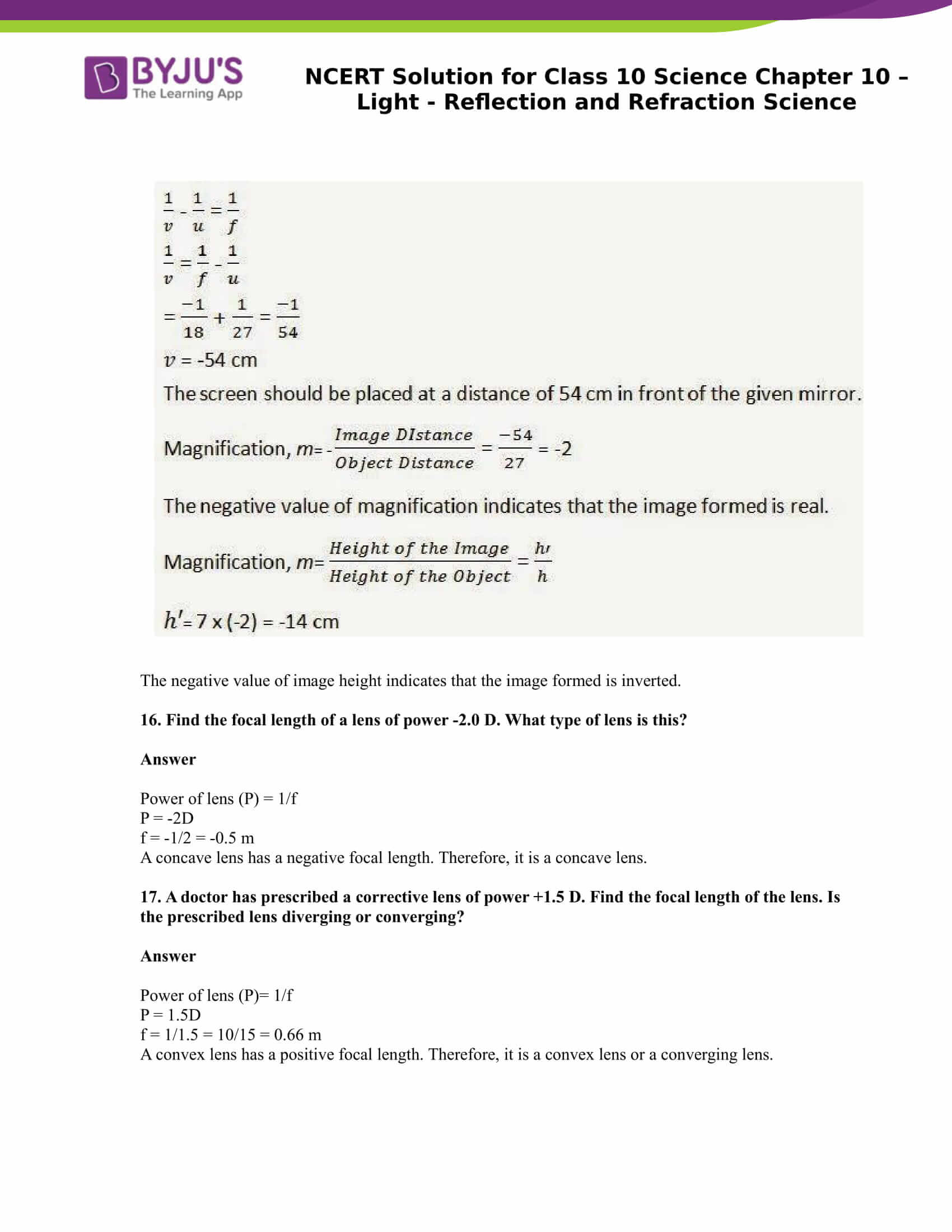## NCERT Solutions For Class 10 Science Chapter 10 – Light Reflection and Refraction

Chapter 10 Light Reflection and Refraction is one of the important chapters in class 10 science and the expected marks weightage of the chapter according to the latest marking scheme is 7 marks. In chapter 10 of class 10 science, students will get well versed with light phenomena such as refraction and reflection. One will learn about spherical mirrors, image formation and to draw ray diagrams.

Topics covered in this chapter:

• Reflection of Light
• Spherical Mirrors
• Image Formation by Spherical Mirrors
• Representation of Images Formed by Spherical Mirrors Using Ray Diagram – 4 Questions ( 2 short, 2 long)
• Mirror Formula and Magnification – 2 Questions (2 Numerical)
• Refraction of Light – 5 Questions ( 1 numerical, 2 Long answers and 1 Short answer)
• Power of Lens – 3 Questions ( 2 Short answers and 1 numerical)

## NCERT Solutions For Class 10 Science Chapter 10 – Light Reflection And Refraction

Have you ever wondered what makes things visible to us? The answer to the question is light. During the day, it is sunlight that helps us see objects. When light falls on an object, it reflects it. This reflected ray when received by our eyes helps us see things. There are numerous wonderful phenomena that are associated with light such as the formation of the rainbow, of stars and many more. In chapter 10 Light Reflection and Refraction of class 10 Science, let us study the phenomena of refraction and reflection using straight-line propagation of light. We shall also try to understand the reflection of light by spherical mirrors in this chapter.

### Key Features of NCERT Solutions for class 10 Science Chapter 10 – Light Reflection and Refraction:

• Provides comprehensive answers to all the questions asked in the chapter
• The language used is lucid and can be understood by all
• The information provided is genuine and appropriate
• These solutions can be referred for CBSE exams, Olympiads and other competitive exams
• Concise answers provided to help students understand better